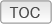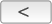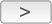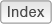Cakewalk // Documentation // SONAR X2 // Scale Velocity dialogDialog box reference ► Scale Velocity dialog

The Process > Scale Velocity command opens the Scale Velocity dialog box, which lets you edit MIDI clips to create crescendos (volume swells) and decrescendos on those instruments that respond to MIDI velocity. Most MIDI instruments map changes in velocity to changes in note loudness. Many synthesizer patches alter the timbre of the sound as well, so that higher velocities produce brighter, as well as louder, sounds.
This command lets you set a starting and ending velocity for the entire time range of the selection. SONAR scales the velocity of each event to create a smooth linear change in velocity. As an option, you can enter a starting and ending percentage; SONAR modifies existing velocity values by the designated percentage.
The Scale Velocity dialog box has the following fields:
Enter a velocity value between 0 and 127 for the first event of the selection, unless you want to use percentages. If you use percentages, enter a number between 0 and the approximate percentage that would create a velocity of 127, when multiplied by the existing velocity of the first event. SONAR rounds off any values you create above 127 to 127.
Enter a velocity value between 0 and 127 for the last event of the selection, unless you want to use percentages. If you use percentages, enter a number between 0 and the approximate percentage that would create a velocity of 127, when multiplied by the existing velocity of the last event. SONAR rounds off any values you create above 127 to 127.